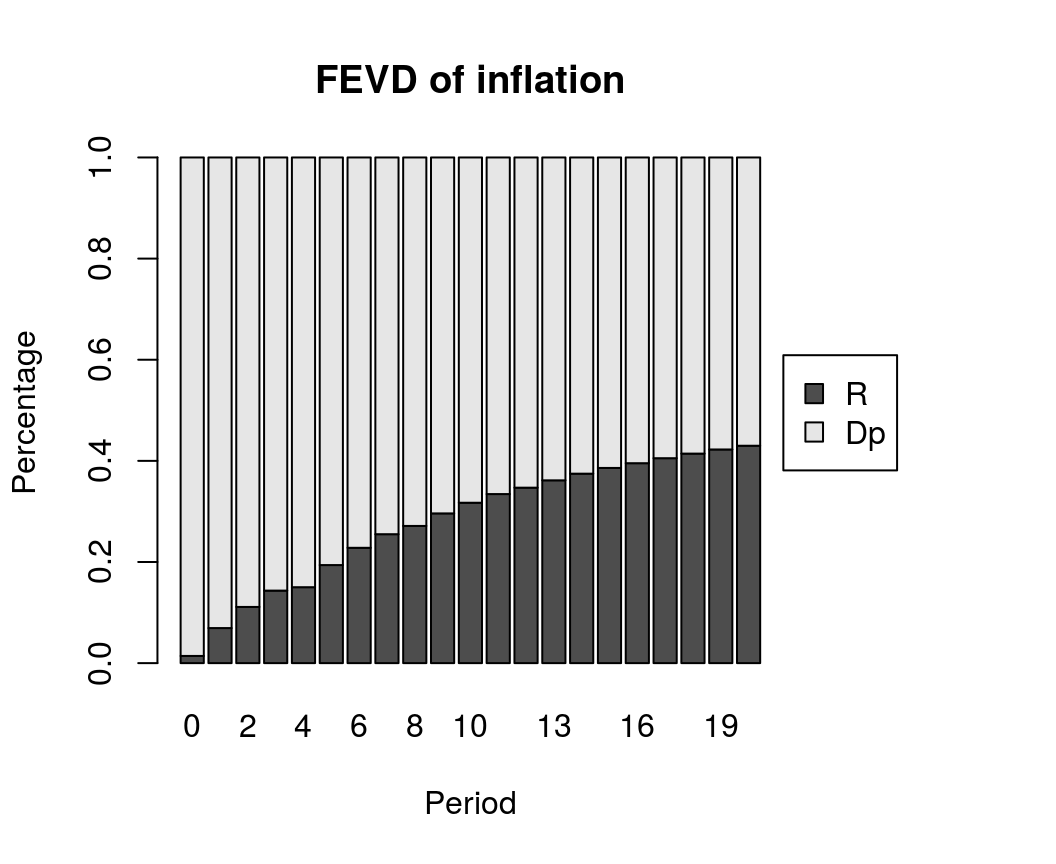# Bayesian Error Correction Models with Priors on the Cointegration Space

with tags r bvec vec cointegration bvartools -

## Introduction

This post provides the code to set up and estimate a basic Bayesian vector error correction (BVEC) model with the bvartools package. The presented Gibbs sampler is based on the approach of Koop et al. (2010), who propose a prior on the cointegration space.

## Data

To illustrate the estimation process, the dataset E6 from Lütkepohl (2007) is used, which contains data on German long-term interest rates and inflation from 1972Q2 to 1998Q4.

library(bvartools)

data("e6")

plot(e6) # Plot the series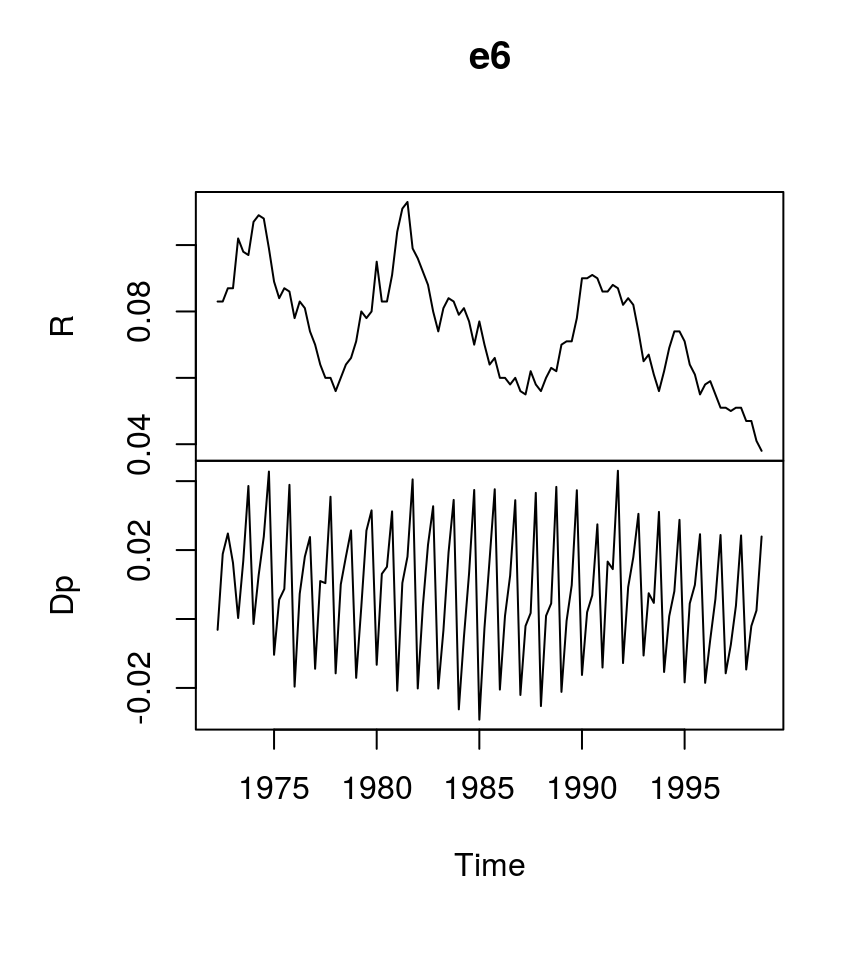The gen_vec function produces the inputs Y, W and X for the BVEC estimator, where Y is the matrix of dependent variables, W is a matrix of potentially cointegrated regressors, and X is the matrix of non-cointegration regressors.

data <- gen_vec(e6, p = 4, const = "unrestricted", season = "unrestricted")

y <- data$Y w <- data$W
x <- data$X ## Estimation # Reset random number generator for reproducibility set.seed(7654321) iter <- 10000 # Number of iterations of the Gibbs sampler burnin <- 5000 # Number of burn-in draws store <- iter - burnin r <- 1 # Set rank t <- ncol(y) # Number of observations k <- nrow(y) # Number of endogenous variables k_w <- nrow(w) # Number of regressors in error correction term k_x <- nrow(x) # Number of differenced regressors and unrestrictec deterministic terms k_alpha <- k * r # Number of elements in alpha k_beta <- k_w * r # Number of elements in beta k_gamma <- k * k_x # Set uninformative priors # ...for non-cointegration parameters a_mu_prior <- matrix(0, k_x * k) # Vector of prior parameter means a_v_i_prior <- diag(0, k_x * k) # Inverse of the prior covariance matrix # ...for cointegration parameters v_i <- 0 p_tau_i <- matrix(0, k_w, k_w) p_tau_i[1:r, 1:r] <- diag(1, r) #...for the error term u_sigma_df_prior <- r # Prior degrees of freedom u_sigma_scale_prior <- diag(0, k) # Prior covariance matrix u_sigma_df_post <- t + u_sigma_df_prior # Posterior degrees of freedom # Initial values beta <- matrix(c(1, -4), k_w, r) u_sigma_i <- diag(.0001, k) u_sigma <- solve(u_sigma_i) g_i <- u_sigma_i # Data containers draws_alpha <- matrix(NA, k_alpha, store) draws_beta <- matrix(NA, k_beta, store) draws_pi <- matrix(NA, k * k_w, store) draws_gamma <- matrix(NA, k_gamma, store) draws_sigma <- matrix(NA, k^2, store) # Start Gibbs sampler for (draw in 1:iter) { # Draw conditional mean parameters temp <- post_coint_kls(y = y, beta = beta, w = w, x = x, sigma_i = u_sigma_i, v_i = v_i, p_tau_i = p_tau_i, g_i = g_i, gamma_mu_prior = a_mu_prior, gamma_V_i_prior = a_v_i_prior) alpha <- temp$alpha
beta <- temp$beta Pi <- temp$Pi
gamma <- temp$Gamma # Draw variance-covariance matrix u <- y - Pi %*% w - matrix(gamma, k) %*% x u_sigma_scale_post <- solve(tcrossprod(u) + v_i * alpha %*% tcrossprod(crossprod(beta, p_tau_i) %*% beta, alpha)) u_sigma_i <- matrix(rWishart(1, u_sigma_df_post, u_sigma_scale_post)[,, 1], k) u_sigma <- solve(u_sigma_i) # Update g_i g_i <- u_sigma_i # Store draws if (draw > burnin) { draws_alpha[, draw - burnin] <- alpha draws_beta[, draw - burnin] <- beta draws_pi[, draw - burnin] <- Pi draws_gamma[, draw - burnin] <- gamma draws_sigma[, draw - burnin] <- u_sigma } } Obtain point estimates as the mean of the parameter draws: Gamma <- rowMeans(draws_gamma) # Obtain means for every row Gamma <- matrix(Gamma, k) # Transform mean vector into a matrix Gamma <- round(Gamma, 3) # Round values dimnames(Gamma) <- list(dimnames(y)[], dimnames(x)[]) # Rename matrix dimensions round(Gamma, 3) # Print ## d.R.1 d.Dp.1 d.R.2 d.Dp.2 d.R.3 d.Dp.3 const season.1 season.2 ## d.R 0.267 -0.187 -0.017 -0.207 0.225 -0.099 0.002 0.001 0.009 ## d.Dp 0.074 -0.383 0.000 -0.421 0.025 -0.361 0.011 -0.034 -0.018 ## season.3 ## d.R 0.000 ## d.Dp -0.017 beta <- rowMeans(t(t(draws_beta) / t(draws_beta)[, 1])) # Obtain means for every row beta <- matrix(beta, k_w) # Transform mean vector into a matrix beta <- round(beta, 3) # Round values dimnames(beta) <- list(dimnames(w)[], NULL) # Rename matrix dimensions beta # Print ## [,1] ## l.R 1.000 ## l.Dp -3.969 Sigma <- rowMeans(draws_sigma) # Obtain means for every row Sigma <- matrix(Sigma, k) # Transform mean vector into a matrix Sigma <- round(Sigma * 10^4, 2) # Round values dimnames(Sigma) <- list(dimnames(y)[], dimnames(y)[]) # Rename matrix dimensions Sigma # Print ## d.R d.Dp ## d.R 0.29 -0.02 ## d.Dp -0.02 0.27 ## bvec objects The bvec function can be used to collect output of the Gibbs sampler in a standardised object, which can be used further for forecasting, impulse response analysis or forecast error variance decomposition. # Number of non-deterministic coefficients k_nondet <- (k_x - 4) * k # Generate bvec object bvec_est <- bvec(y = y, w = w, x = x, Pi = draws_pi, Gamma = draws_gamma[1:k_nondet,], C = draws_gamma[(k_nondet + 1):nrow(draws_gamma),], Sigma = draws_sigma) Posterior draws can be thinned with function thin: bvec_est <- thin(bvec_est, thin = 5) The function bvec_to_bvar can be used to transform the VEC model into a VAR in levels: bvar_form <- bvec_to_bvar(bvec_est) ## Forecasts bvar_pred <- predict(bvar_form, n.ahead = 10, new_D = t(bvar_form$x[9:12, 91:100]))

plot(bvar_pred)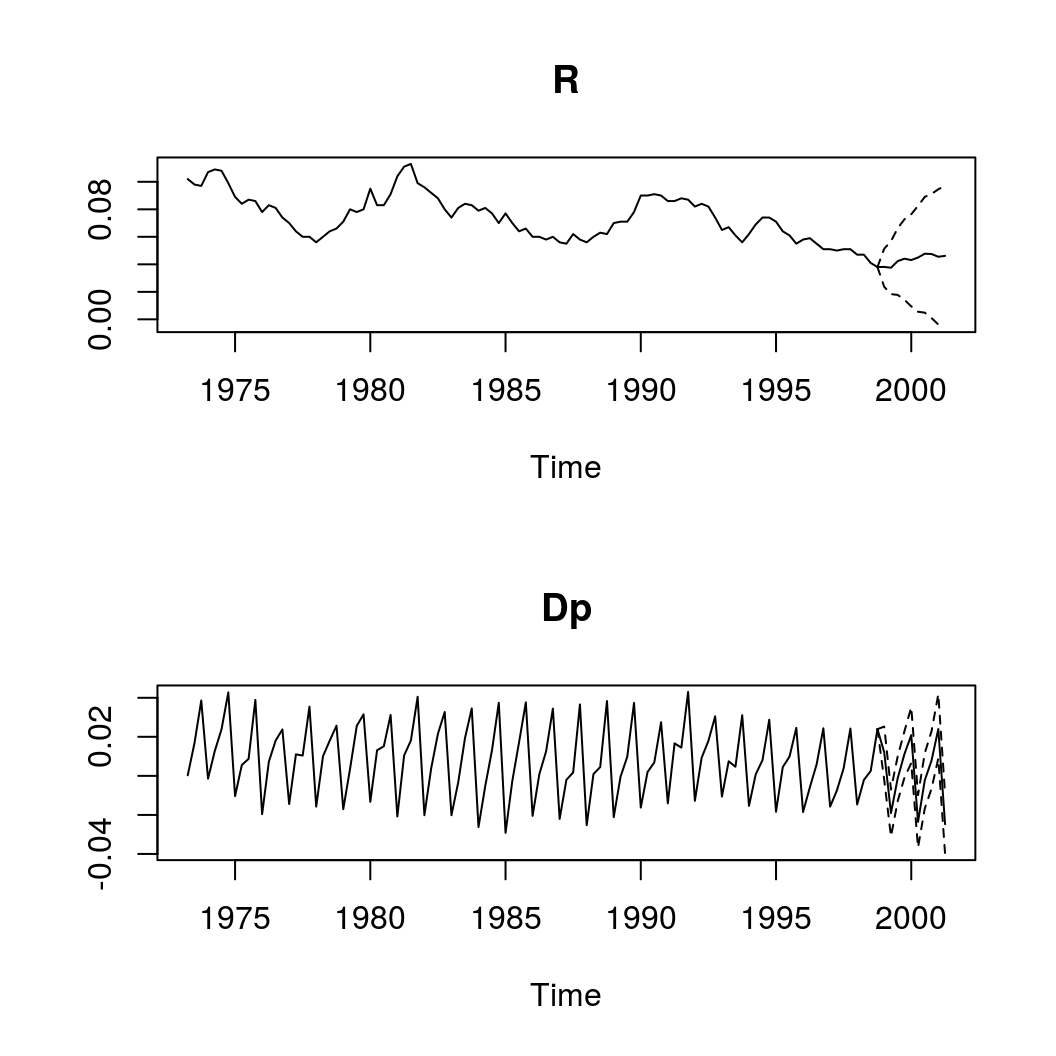## Impulse response analysis

Impulse responses for VECs can be constructed from their VAR respresentations.

IR <- irf(bvar_form, impulse = "R", response = "Dp", n.ahead = 20)

plot(IR, main = "Forecast Error Impulse Response", xlab = "Year", ylab = "Response")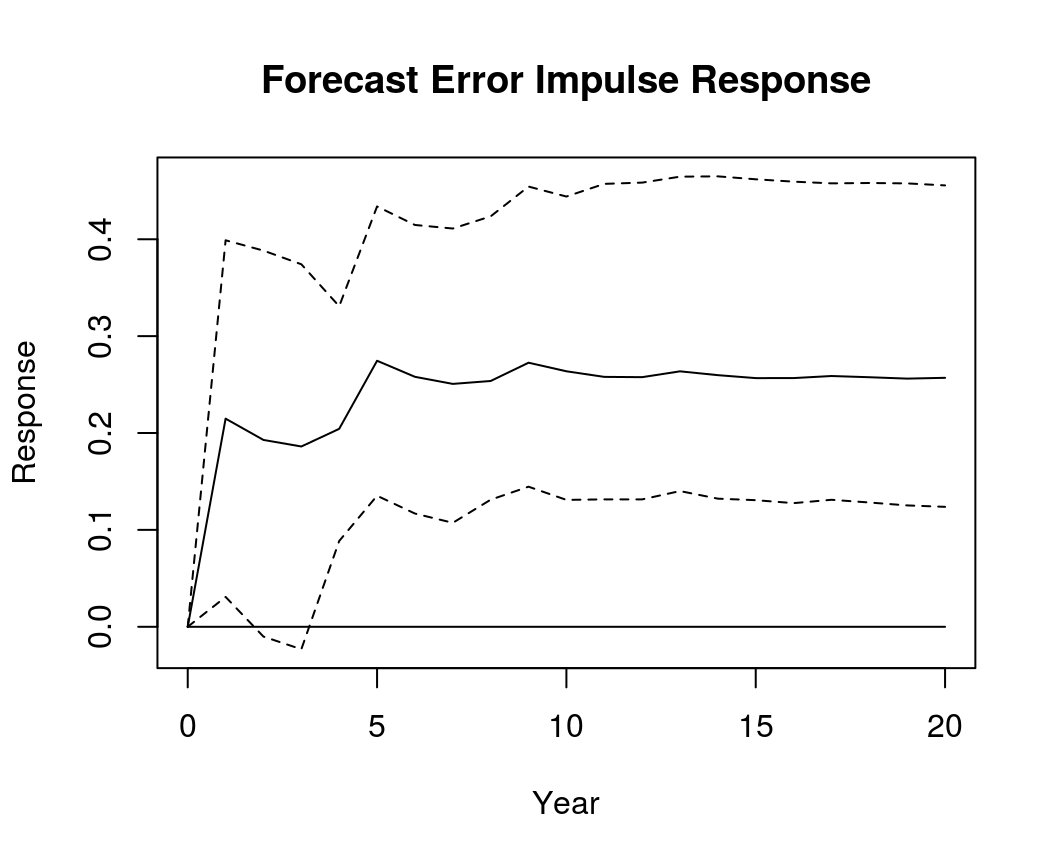### Orthogonalised impulse response

OIR <- irf(bvar_form, impulse = "R", response = "Dp", n.ahead = 20, type = "oir")

plot(OIR, main = "Orthogonalised Impulse Response", xlab = "Year", ylab = "Response")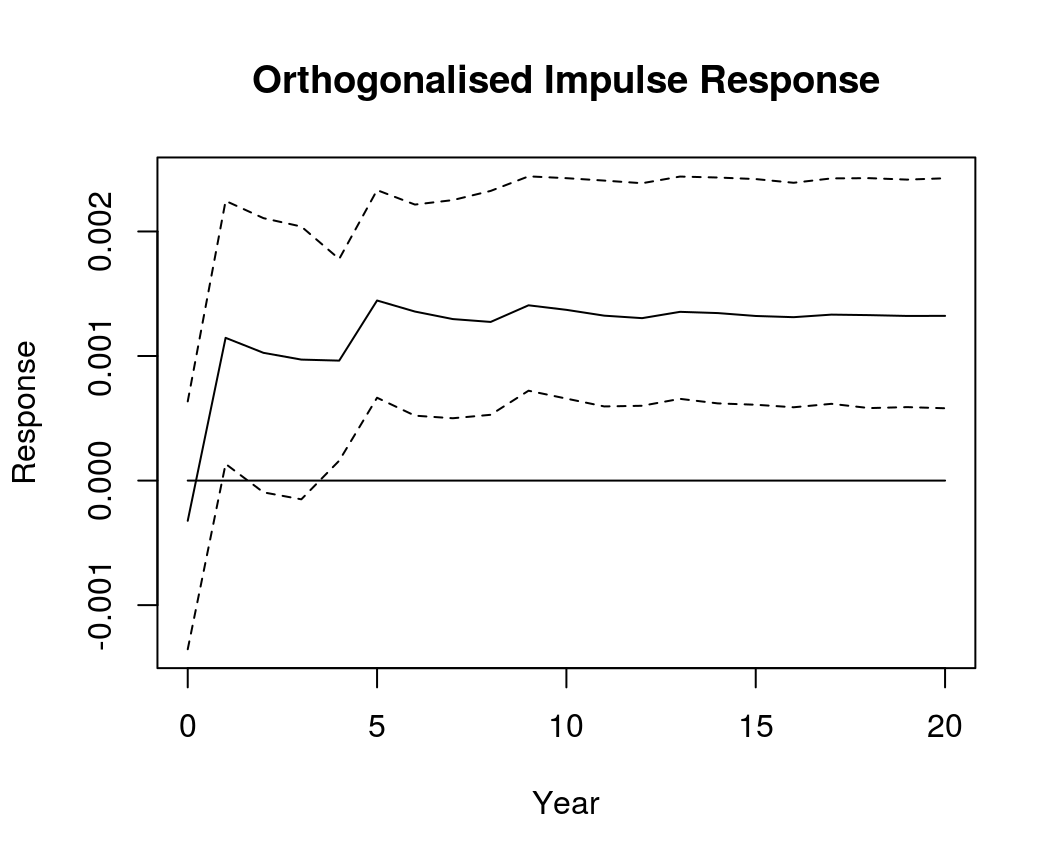### Generalised impulse response

GIR <- irf(bvar_form, impulse = "R", response = "Dp", n.ahead = 20, type = "gir")

plot(GIR, main = "Generalised Impulse Response", xlab = "Year", ylab = "Response")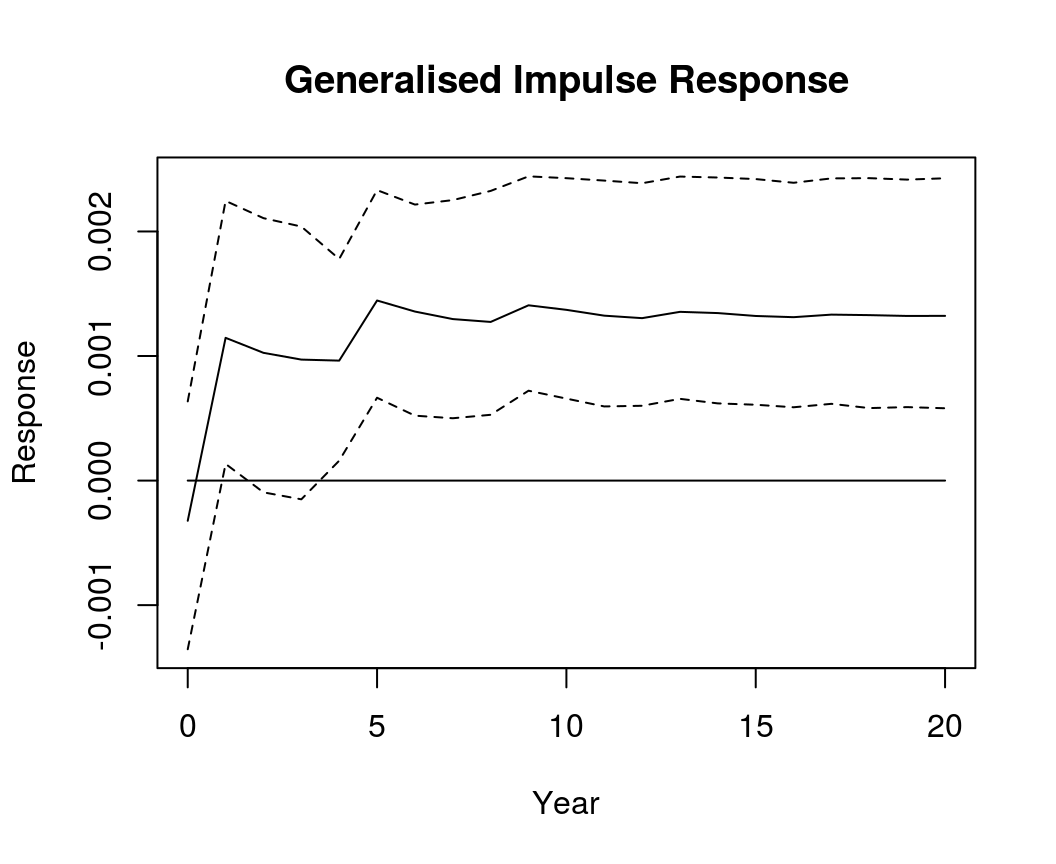### Forecast error variance decomposition

bvec_fevd <- fevd(bvar_form, response = "Dp", n.ahead = 20)

plot(bvec_fevd, main = "FEVD of inflation")